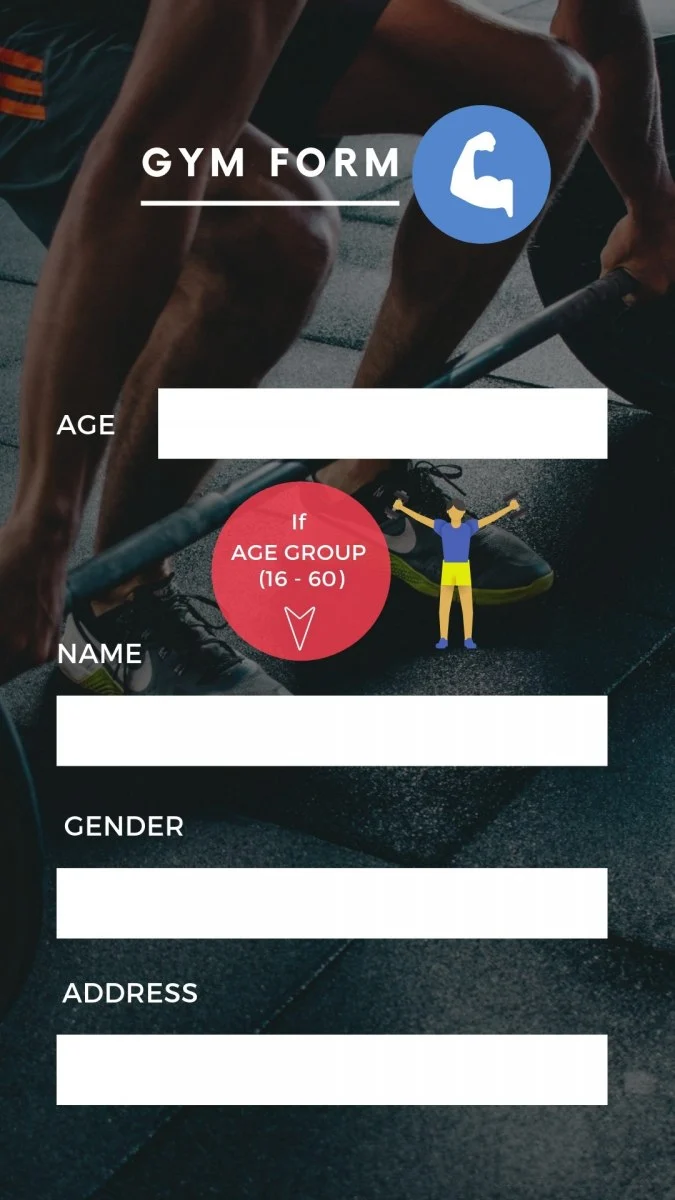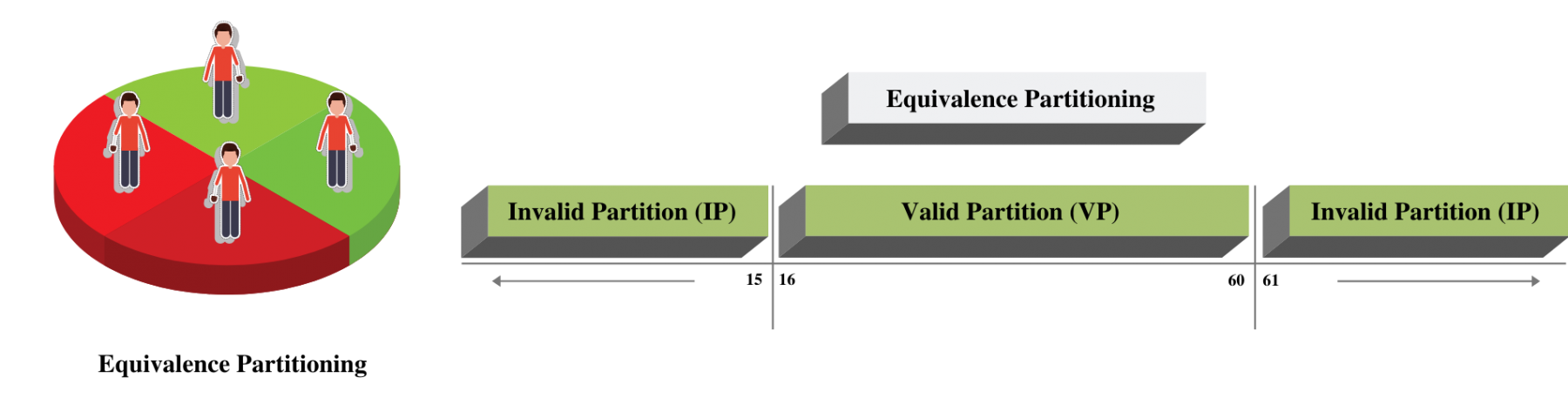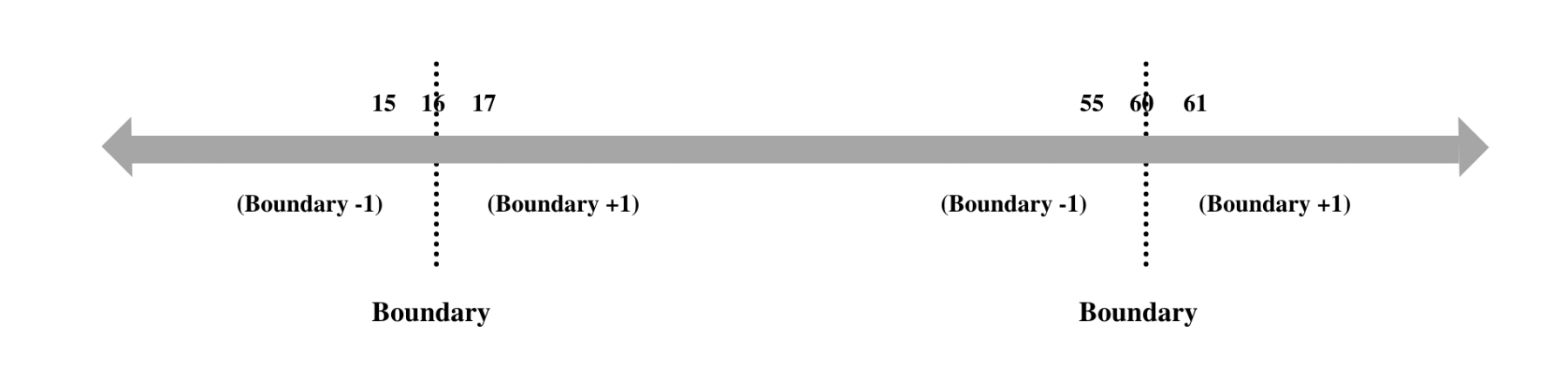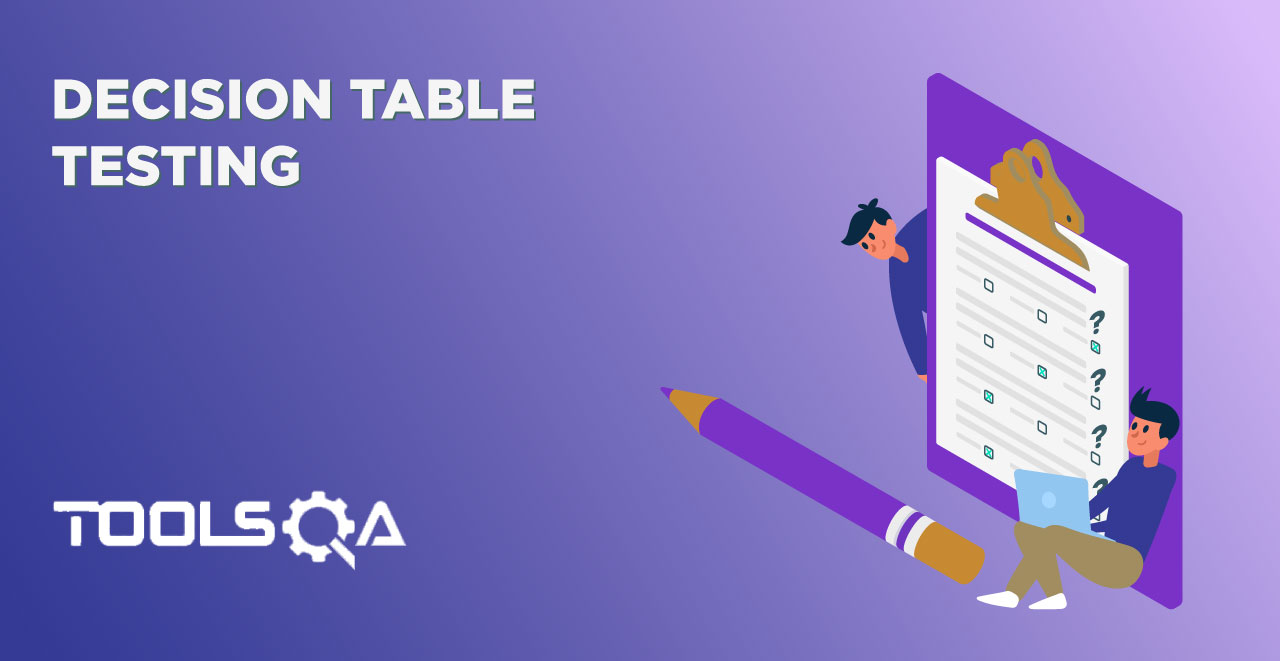We already know that Black box testing involves validating the system without knowing its internal design. We have also discussed the pitfalls of Equivalence partitioning and how they can fail at partition boundaries. In case you haven't read our article on Equivalence Partition, I would highly recommend to read it before you read this one. In this article, we will discuss another black box testing technique known as Boundary Value Analysis. We will also see how this technique compliments Equivalence partitioning.

• What is Boundary Value Analysis?
• How to do Boundary Value Analysis?
• Boundary Value Analysis with Equivalence Partitioning
• Pitfalls of Boundary value Analysis

## What is Boundary Value Analysis?

The basis of Boundary Value Analysis (BVA) is testing the boundaries at partitions (Remember Equivalence Partitioning !). BVA is an extension of equivalence partitioning. However, this is useable only when the partition is ordered, consisting of numeric or sequential data. The minimum and maximum values of a partition are its boundary values.

We have seen that there are high chances of finding the defects at the boundaries of a partition (E.g., A developer using >10 instead of >= 10 for a condition). Equivalence partitioning alone was not sufficient to catch such defects. Therefore, a need to define a new technique that can detect anomalies at the boundaries of the partition arose. It is how Boundary value analysis came into the picture.

Boundary value analysis can perform at all test levels, and its primarily used for a range of numbers, dates, and time.

### How to Do Boundary Value Analysis?

Now that we have got some idea on boundary value analysis let's understand how to derive test conditions using this technique. We will refer to the same example of gym form (Refer to our article on Equivalence Partitioning) where we need to enter Age.The first step of Boundary value analysis is to create Equivalence Partitioning, which would look like below.Now Concentrate on the Valid Partition, which ranges from 16-60. We have a 3 step approach to identify boundaries:• Identify Exact Boundary Value of this partition Class - which is 16 and 60.
• Get the Boundary value which is one less than the exact Boundary - which is 15 and 59.
• Get the Boundary Value which is one more than the precise Boundary - which is 17 and 61 . If we combine them all, we will get below combinations for Boundary Value for the Age Criteria.

Valid Boundary Conditions : Age = 16, 17, 59, 60

Invalid Boundary Conditions : Age = 15 61,

It's straightforward to see that valid boundary conditions fall under Valid partition class, and invalid boundary conditions fall under Invalid partition class.

Can you figure out why we have not used 16.1, 15.9, 59.9, and 60.1 as the boundary increment and decrement values? It's a concept that has an insufficient explanation in most of the articles. Therefore, let's take another example to explain this. Assume that you are entering your weight on a website. Based on your weight and height, the site will tell you the Body Mass Index (BMI). You can enter values from 30 to 150 kg in the weight input field. The weight input field only allows natural numbers i.e., positive integers!

In this case, if you will create the boundaries using the same method - you will end up with

Valid Boundary Conditions : Age = 30, 31, 149, 150

Invalid Boundary Conditions : Age = 29, 151

Now consider the same scenario, but the weight input field allows decimal numbers up to 1 decimal place. In this case, the boundary conditions will come as:

Valid Boundary Conditions : Age = 30, 30.1, 149.9, 150

Invalid Boundary Conditions : Age = 29.9, 150.1

Did you see the difference? We take the minimal acceptable value on either side of the boundary. If we take the value as 30.01, then we end up testing the software for two decimals where the requirement is one decimal place. It is a separate test condition and should not be mixed up with Boundary value.

Measurement of the Boundary coverage for a partition can happen as the number of boundary values tested divided by the total number of boundary test values identified.

### Boundary Value Analysis with Equivalence Partitioning

We have got a fair understanding of Boundary Value Analysis now. So, let's see how we can combine it with Equivalence partitioning to get a full set of test conditions.

Coming back to our earlier example, let's review the diagram again.The range is from 16 - 60, and Boundary Value analysis gives us test conditions as 15, 16, 17, 59, 60, 61. If you have a close look, don't you think we have already covered Valid Equivalence partitioning by covering up 17, 59, and Invalid Equivalence Partitioning by covering 15 and 61? After all Equivalence partitioning says that we should choose a number between 16-60 for valid partition and less than 16 or more than 60 for invalid partition. So, if the boundary value is already covering Equivalence partitioning, why do we need partitioning as a separate technique? It is a concept that is not clear to most of the folks, and not many articles have explained it clearly.

Theoretically, Boundary value has indeed covered Equivalence partition, but we still need a partition. If we only apply Boundary value, and it fails, we will never know whether the edge condition failed, or the entire partition failed. Let's comprehend it with the help of an example. Continuing with our gym form, let's assume the developer has written below logic :

If (age < = 17 ) Then Don't allow Gym Membership

If (age > 60) Then Don't allow Gym Membership

If you look at the logic, you will realize that the logic should have been If (age <17), but the developer added = wrong sign. Did you also realize that the logic for the entire valid partition is missing? If (age>=16 and age <= 60 ) Then allow Gym membership !

If we only use boundary condition value 17, it will fail the test execution. However, it will not tell you whether the boundary condition failed or if the entire partition failed. As such, it's essential to use an Equivalence partition value, which is not a boundary value. In this case, if we use the value 20, it will fail the execution. It will give a clear indication that the developer has missed implementing the entire partition.

So if we combine both Boundary Value and Equivalence Partitioning, our test conditions will be :

Valid Boundary Conditions : Age = 16, 17, 59, 60

Invalid Boundary Conditions : Age = 15, 61

Valid Equivalence Partition : Age = 25

Invalid Equivalence Partition : Age = 5 , 65

### Pitfalls of BVA

After applying both boundary value and Equivalence partitioning, can we confidently say that we got all the required coverage? Unfortunately, it's not that simple! Boundary value and equivalence partitioning assume that the application will not allow you to enter any other characters or values. Such characters, like @ or negative values or even alphabets, will not be allowed to enter. This assumption is, however, not valid for all applications, and it's essential to test these out before we can say that the field value is completely working.

Apart from that, we can have situations where the input value depends on the decision of another value. E.g., If the Gym form has another field Male and Female, and the age limit will vary according to that selection. Boundary value alone cannot handle such variations, and this leads us to another black box technique called Decision Table Testing. We will discuss that in detail in our next article. Stay tuned!ReviewersVirender Singh